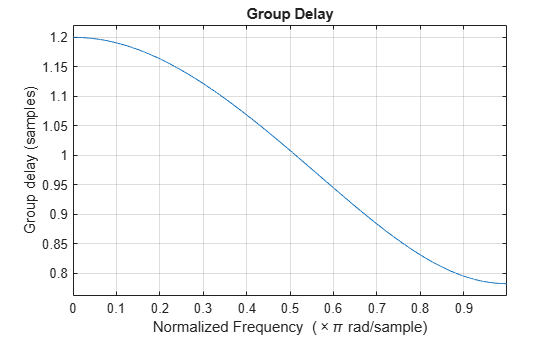Main Content

# fdesign.fracdelay

Fractional delay filter specification object

## Syntax

```d = fdesign.fracdelay(delta) d = fdesign.fracdelay(delta,'N') d = fdesign.fracdelay(delta,'N',n) d = fdesign.fracdelay(delta,n) d = fdesign.fracdelay(...,fs) ```

## Description

`d = fdesign.fracdelay(delta)` constructs a default fractional delay filter designer `d` with the filter order set to 3 and the delay value set to `delta`. The fractional delay `delta` must be between 0 and 1 samples.

`d = fdesign.fracdelay(delta,'N')` initializes the filter designer specification to `N`, where `N` specifies the fractional delay filter order and defaults to filter order of 3.

Use `designmethods``(d)` to get a list of the design methods available for a specification.

`d = fdesign.fracdelay(delta,'N',n)` initializes the filter designer to `N` and sets the filter order to `n`.

`d = fdesign.fracdelay(delta,n)` assumes the default specification `N`, filter order, and sets the filter order to the value you provide in input `n`.

`d = fdesign.fracdelay(...,fs)` adds the argument `fs`, specified in units of Hertz (Hz) to define the sampling frequency. In this case, specify the fractional delay `delta` to be between 0 and 1/`fs`.

## Examples

collapse all

Design a second-order fractional delay filter of 0.2 samples using the Lagrange method. Implement the filter using a Farrow fractional delay (fd) structure.

```d = fdesign.fracdelay(0.2,'N',2); secondOrderFrac = design(d,'lagrange','filterstructure','farrowfd'); fvtool(secondOrderFrac,'analysis','grpdelay')```Design a cubic fractional delay filter with a sampling frequency of 8 kHz and fractional delay of 50 microseconds using the Lagrange method.

```d = fdesign.fracdelay(50e-6,'N',3,8000); cubicFrac = design(d, 'lagrange', 'FilterStructure', 'farrowfd');```

## See Also

Introduced in R2011a

## Support